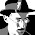## Sunday, November 30, 2014

### Change in temperature in Netherlands over the last century

I read a post 'race for the warmest year' at sargasso.nl. They used a plot, originating from Ed Hawkins to see how 2014 progressed to be warmest year. Obviously I wanted to make the same plot using R. In addition, I wondered which parts of the year had most increased in temperature.

### Data

Similar to last week, data are acquired from KNMI. They have various sets of data, this page has a selection form which leads to the data used today. The data comes with a header explaining details, unfortunately in Dutch. Of relevance for this post is TG, average and minimum temperature in 0.1 C. Station used is de Bilt, where they got most data. Prior to reading the data into R, the explanatory text header was removed.
The data input is completed by converting YYYYMMDD to date, year, month and dayno variables. Prior to analysis for simplicity a leap day was removed. I chose merge() rather than a dplyr merge function since the latter releveled my moth factor. The data.frame mylegend is used later on to label the first of the month.
library(plyr)
library(dplyr)
library(ggplot2)
library(locfit)
library(plotrix)
Sys.setlocale(category = "LC_TIME", locale = "C")
r2 <- mutate(r1,
date = as.Date(format(YYYYMMDD),'%Y%m%d'),
month =factor(months(date,abbreviate=TRUE),
levels=months(as.Date(
paste('2014',
formatC(1:12,digits=2,width=2,flag='0'),
'01',sep='-')),
abbreviate=TRUE)),
yearf=factor(format(date,'%Y')),
yearn=as.numeric(substr(YYYYMMDD,1,4)),
day=format(date,'%e'))

days <- filter(r2,yearn==1901) %>%
mutate(.,dayno=format(date,'%j') ) %>%
select(.,month,day,dayno)

r3 <- merge(r2,days,all=TRUE) %>%
filter(.,!grepl('0229',YYYYMMDD)) %>%
mutate(.,daynon=as.numeric(dayno))

mylegend <- filter(days,day==' 1') %>%
mutate(.,daynon=as.numeric(dayno))

### Plots

#### Cumulative average by year

Each line is a separate year. For this plot is is needed to have year a factor. Unfortunately, I was not able to get a colourbar legend for colors, that required a continuous year variable. Green is beginning last century, pinkish is recent, the fat black line is 2014.

r4 <- group_by(r3,yearf) %>%
mutate(.,cmtemp = cummean(TG/10))

g1 <- ggplot(r4,aes(x=daynon,y=cmtemp,
col=yearf))
g1 + geom_line(alpha=.4,show_guide=FALSE)  +
scale_x_continuous('Day',
breaks=mylegend\$daynon,
labels=mylegend\$month,
expand=c(0,0)) +
scale_y_continuous('Temperature (C)')  +
geom_line(data=r4[r4\$yearf=='2014',],
aes(x=daynon,y=cmtemp),
col='black',
size=2)

#### 2014 with average of 30 years

To get a better idea how 2014 compares to previous years, the average of 30 years has been added. We had warm year, except for August, which suggested an early spring. In hindsight, second half of August had colder days than beginning April or end October.

r3\$Period <- cut(r3\$yearn,c(seq(1900,2013,30),2013,2014),
labels=c('1901-1930','1931-1960',
'1961-1990','1991-2013','2014'))
g1 <- ggplot(r3[r3\$yearn<2014,],aes(x=daynon,y=TG/10,col=Period))
g1 + geom_smooth(span=.15,method='loess',size=1.5)  +
scale_x_continuous('Day',
breaks=mylegend\$daynon,
labels=mylegend\$month,
expand=c(0,0)) +
geom_line(#aes(x=daynon,y=TG/10),
data=r3[r3\$yearn==2014,]) +
scale_y_continuous('Temperature (C)')

#### Change by year

Finally, a plot showing how temperature changed within the years. To obtain this plot, I needed a day corrected base temperature. The baseline temperature is smoothed over days for years 1901 to 1924. The baseline was used to get a corrected baseline, which was subsequently smoothed over years and days.
Smoothers have edge effects, to remove these from the visual part, January and December have been added as extra to the data. Hence within the year there are only minimal edge effects.
The plot shows that middle last century, some parts of the year actually had a drop in temperature. In contrast, November has gradually been getting warmer since middle last century. The new century has seen quite an increase.
myyears <- r3[r3\$yearn<1925,]
m13 <- filter(myyears,daynon<30) %>%
mutate(.,daynon=daynon+365)
m0 <- filter(myyears,daynon>335) %>%
mutate(.,daynon=daynon-365)
myyears <- rbind_list(m0,myyears,m13)

nn <- .2
mymod <- locfit(TG ~ lp(daynon,nn=nn),
data=myyears)
topred <- data.frame(daynon=1:365)
topred\$pp <- predict(mymod,topred)
#plot(pp~ daynon,data=topred)

r5 <- merge(r3,topred) %>%
mutate(.,tdiff=(TG-pp)/10) %>%
select(.,tdiff,daynon,yearn)
m13 <- filter(r5,daynon<30) %>%
mutate(.,daynon=daynon+365,
yearn=yearn-1)
m0 <- filter(r5,daynon>335) %>%
mutate(.,daynon=daynon-365,
yearn=yearn+1)
r6 <- rbind_list(m0,r5,m13)

topred <- expand.grid(
daynon=seq(1:365),
yearn=1901:2014)
topred\$pp2 <- locfit(
tdiff ~ lp(yearn,daynon,nn=nn),
data=r6) %>%
predict(.,topred)
#topred <- arrange(topred,daynon,yearn)

myz <- matrix(topred\$pp2,ncol=365)
zmin <- floor(min(topred\$pp2)*10)/10
zmax <- ceiling(max(topred\$pp2)*10)/10
myseq <- seq(zmin,zmax,.1)
par(mar=c(5,4,4,6))
image(myz,useRaster=TRUE,
axes=FALSE,frame.plot=TRUE,
col=colorRampPalette(c('blue','red'))(length(myseq)-1),
breaks=myseq)
axis((seq(10,114,by=10)-1)/113,labels=seq(1910,2010,by=10),side=1)
axis((mylegend\$daynon-1)/365,labels=mylegend\$month,side=2)
rect.col=colorRampPalette(c('blue','red'))(length(myseq)-1))

1.Nice work. What about put the txt file on github?
Thanks & Best regards
Raffaele Marrese - Environment Agency - Italy Apulia
r.marrese@gmail.com

2.I will really appreciate if you could share your .txt.

Best

Alex